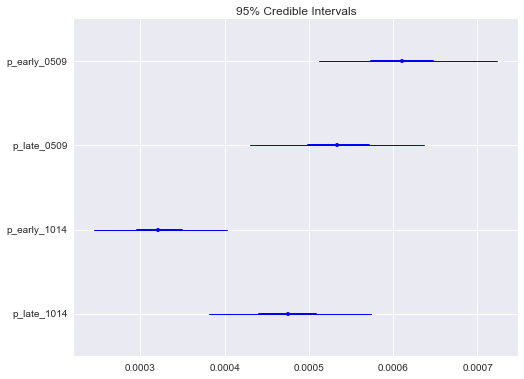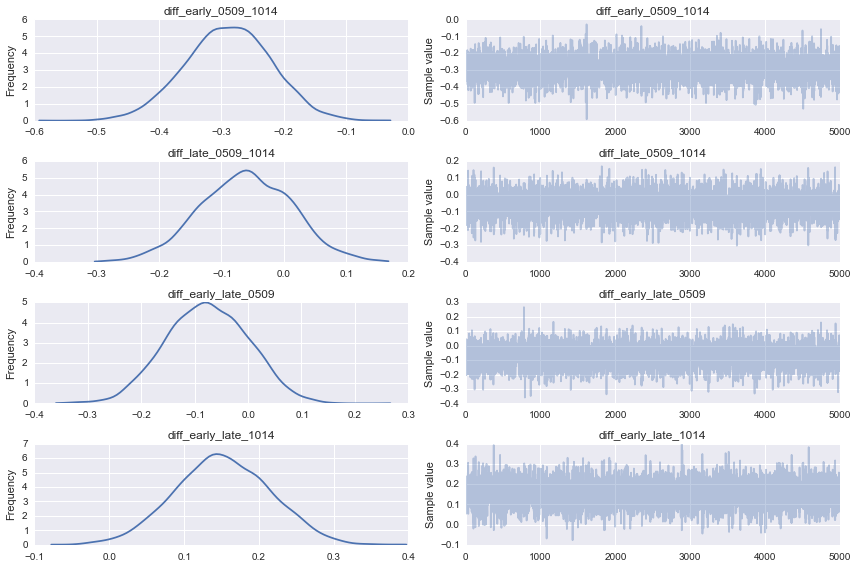In :
%matplotlib inline
import numpy as np
import seaborn as sns
sns.set()
from pymc3 import *

np.random.seed(42)


Extract data

In :
data = np.reshape([2005, 52, 30, 21, 1, 40280, 1.29, 0.74, 0.52, 2006, 55, 33, 19, 3, 42615, 1.29, 0.77, 0.45,
2007, 46, 25, 21, 0, 43199, 1.06, 0.58, 0.49, 2008, 52, 21, 22, 9, 43237, 1.20, 0.49, 0.51,
2009, 52, 19, 29, 4, 41476, 1.25, 0.46, 0.70, 2010, 35, 14, 20, 1, 40147, 0.87, 0.35, 0.50,
2011, 32, 14, 18, 0, 40366, 0.79, 0.35, 0.45, 2012, 40, 11, 23, 6, 40571, 0.99, 0.27, 0.57,
2013, 33, 14, 17, 2, 40792, 0.81, 0.34, 0.42, 2014, 34, 12, 18, 4, 41788, 0.81, 0.29, 0.43],
(10,9))

In :
year, total, early, late, late_late, births, *rates = data.T.astype(int)


Build binomial model for GBS

In :
with Model() as simple_model:

# Probabilities for 2005-2009
p_early_0509 = Beta('p_early_0509', 1, 1)
p_late_0509 = Beta('p_late_0509', 1, 1)

# Difference between early and late in 2005-2009
diff_early_late_0509 = Deterministic('diff_early_late_0509', 1000*(p_late_0509 - p_early_0509))

# Probabilities for 2010-2014
p_early_1014 = Beta('p_early_1014', 1, 1)
p_late_1014 = Beta('p_late_1014', 1, 1)

# Difference between early and late in 2010-2014
diff_early_late_1014 = Deterministic('diff_early_late_1014', 1000*(p_late_1014 - p_early_1014))

# Differences between periods within early group
diff_early_0509_1014 = Deterministic('diff_early_0509_1014', 1000*(p_early_1014 - p_early_0509))
# Differences between periods within late group
diff_late_0509_1014 = Deterministic('diff_late_0509_1014', 1000*(p_late_1014 - p_late_0509))

pre_2010 = year < 2010

# Binomial likelihoods
early_0509 = Binomial('early_0509', births[pre_2010], p_early_0509, observed=early[pre_2010])
late_0509 = Binomial('late_0509', births[pre_2010], p_late_0509, observed=late[pre_2010])
early_1014 = Binomial('early_1014', births[~pre_2010], p_early_1014, observed=early[~pre_2010])
late_1014 = Binomial('late_1014', births[~pre_2010], p_late_1014, observed=late[~pre_2010])



Run the model using Hamiltonian MC

In :
with simple_model:

start = find_MAP()
step = NUTS(scaling=start)
trace = sample(5000, step, start)

 [-----------------100%-----------------] 5000 of 5000 complete in 6.3 sec

Estimates of binomial probabilities

In :
forestplot(trace, vars=['p_early_0509', 'p_late_0509', 'p_early_1014', 'p_late_1014']);Estimates of differences between probabilities

In :
summary(trace, vars=[diff_early_late_0509, diff_early_late_1014, diff_early_0509_1014, diff_late_0509_1014])

diff_early_late_0509:

Mean             SD               MC Error         95% HPD interval
-------------------------------------------------------------------

-0.076           0.077            0.001            [-0.227, 0.067]

Posterior quantiles:
2.5            25             50             75             97.5
|--------------|==============|==============|--------------|

-0.224         -0.130         -0.077         -0.023         0.071

diff_early_late_1014:

Mean             SD               MC Error         95% HPD interval
-------------------------------------------------------------------

0.152            0.064            0.001            [0.032, 0.278]

Posterior quantiles:
2.5            25             50             75             97.5
|--------------|==============|==============|--------------|

0.028          0.109          0.151          0.196          0.275

diff_early_0509_1014:

Mean             SD               MC Error         95% HPD interval
-------------------------------------------------------------------

-0.289           0.069            0.001            [-0.421, -0.155]

Posterior quantiles:
2.5            25             50             75             97.5
|--------------|==============|==============|--------------|

-0.426         -0.335         -0.288         -0.242         -0.159

diff_late_0509_1014:

Mean             SD               MC Error         95% HPD interval
-------------------------------------------------------------------

-0.060           0.073            0.001            [-0.217, 0.072]

Posterior quantiles:
2.5            25             50             75             97.5
|--------------|==============|==============|--------------|

-0.206         -0.111         -0.060         -0.008         0.084



### Conclusions¶

There is a 95% probability the true difference between early rates in 2010-2014 versus 2005-2009 is between -0.421 and -0.155, with an estimated median difference of -0.288. This interval does not contain zero, so we are sure that the rate in 2010-14 is smaller than the rate in 2005-09.

There is a 95% probability the true difference between late rates in 2010-2014 versus 2005-2009 is between -0.217 and 0.072, with an estimated median difference of -0.060. This interval contains zero, so we are not confident that the estimated difference is real.

There is a 95% probability the true difference between early rate versus late rate in 2005-2009 is between -0.227 and 0.067, with an estimated median difference of -0.077. This interval contains zero, so we are not confident that the estimated difference is real.

There is a 95% probability the true difference between early rate versus late rate in 2010-2014 is between 0.032 and 0.278, with an estimated median difference of 0.151. This interval does not contain zero, so we are sure that the late rate is larger than the early rate in 2010-14.

In :
traceplot(trace, vars=['diff_early_0509_1014', 'diff_late_0509_1014',
'diff_early_late_0509', 'diff_early_late_1014']);In :
n_white = 327017.2
n_black = 70874.54
eos_white = 148
los_white = 96
eos_black = 221
los_black = 132

In :
with Model() as race_model:

p_eos_white = Beta('p_eos_white', 1, 1)
p_los_white = Beta('p_los_white', 1, 1)
p_eos_black = Beta('p_eos_black', 1, 1)
p_los_black = Beta('p_los_black', 1, 1)

eos_diff = Deterministic('eos_diff', 1000*(p_eos_white - p_eos_black))
los_diff = Deterministic('los_diff', 1000*(p_los_white - p_los_black))

y_eos_white = Binomial('y_eos_white', n_white, p_eos_white, observed=eos_white)
y_los_white = Binomial('y_los_white', n_white, p_los_white, observed=eos_white)
y_eos_black = Binomial('y_eos_black', n_black, p_eos_black, observed=eos_black)
y_los_black = Binomial('y_los_black', n_black, p_los_black, observed=eos_black)

In :
with race_model:
start = find_MAP()
step = NUTS(scaling=start)
race_trace = sample(5000, step, start)

 [-----------------100%-----------------] 5000 of 5000 complete in 3.3 sec
In :
summary(race_trace, vars=['eos_diff', 'los_diff'])

eos_diff:

Mean             SD               MC Error         95% HPD interval
-------------------------------------------------------------------

-2.672           0.223            0.003            [-3.105, -2.239]

Posterior quantiles:
2.5            25             50             75             97.5
|--------------|==============|==============|--------------|

-3.124         -2.817         -2.671         -2.519         -2.247

los_diff:

Mean             SD               MC Error         95% HPD interval
-------------------------------------------------------------------

-2.678           0.225            0.003            [-3.135, -2.258]

Posterior quantiles:
2.5            25             50             75             97.5
|--------------|==============|==============|--------------|

-3.132         -2.828         -2.671         -2.520         -2.252